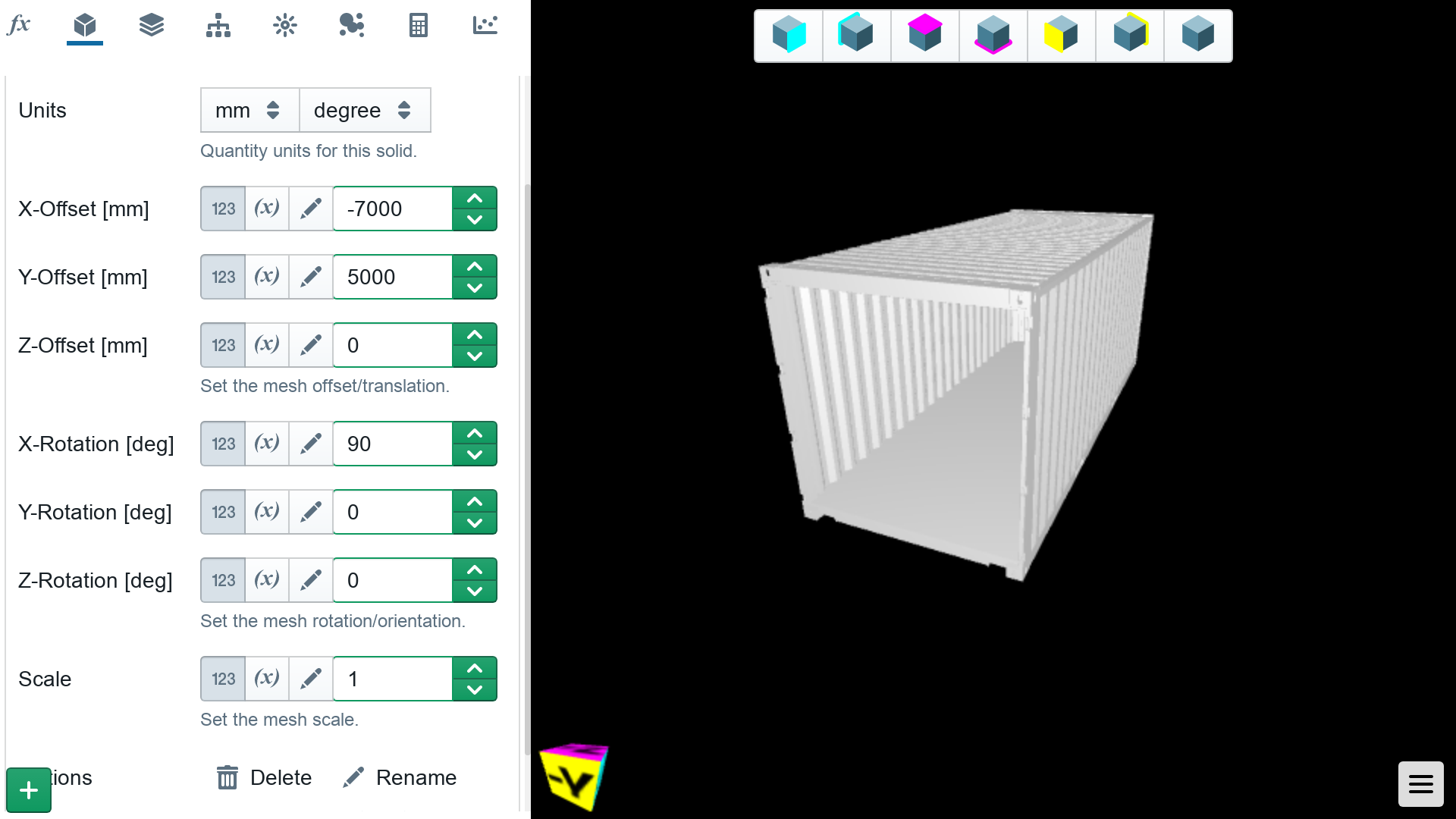# Shapes

This section describes the geometry controls in Shielding Studio for describing your environment.

## Box

Boxes are just that. Rectangular prisms with positive dimensions in the x-, y-, and z-directions. Length controls the dimension the x-direction, width controls the dimension in the y-direction, and height controls the dimension in the z-direction.

#### Parameters

• `length > 0`
• `width > 0`
• `height > 0`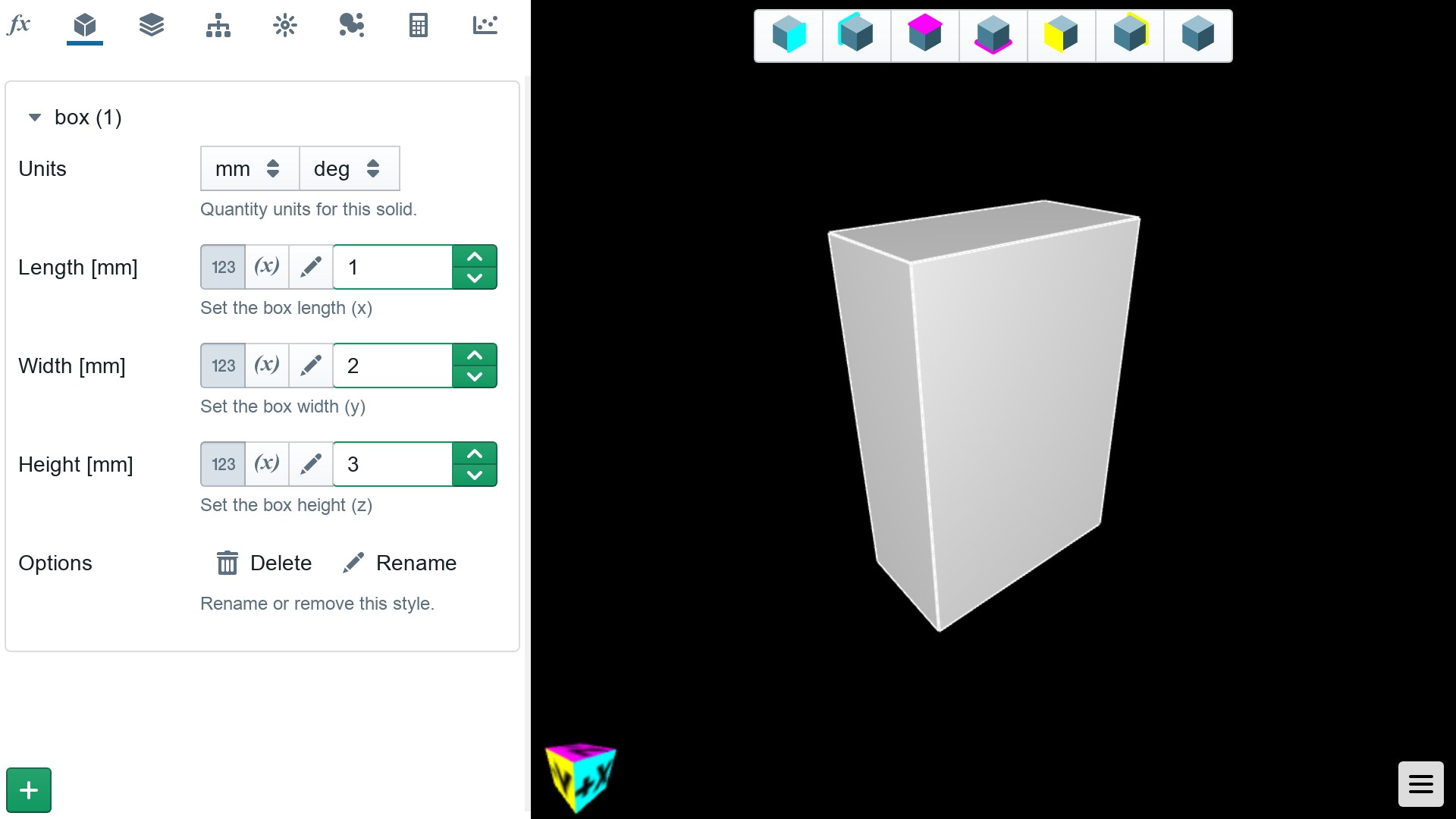## Cone

The basic cone is defined with a height, and upper and lower radii. The upper radius can be equal to zero, to define a pointy cone.

#### Parameters

• `height > 0`

• `upper outer radius >= 0`

• `lower outer radius > 0`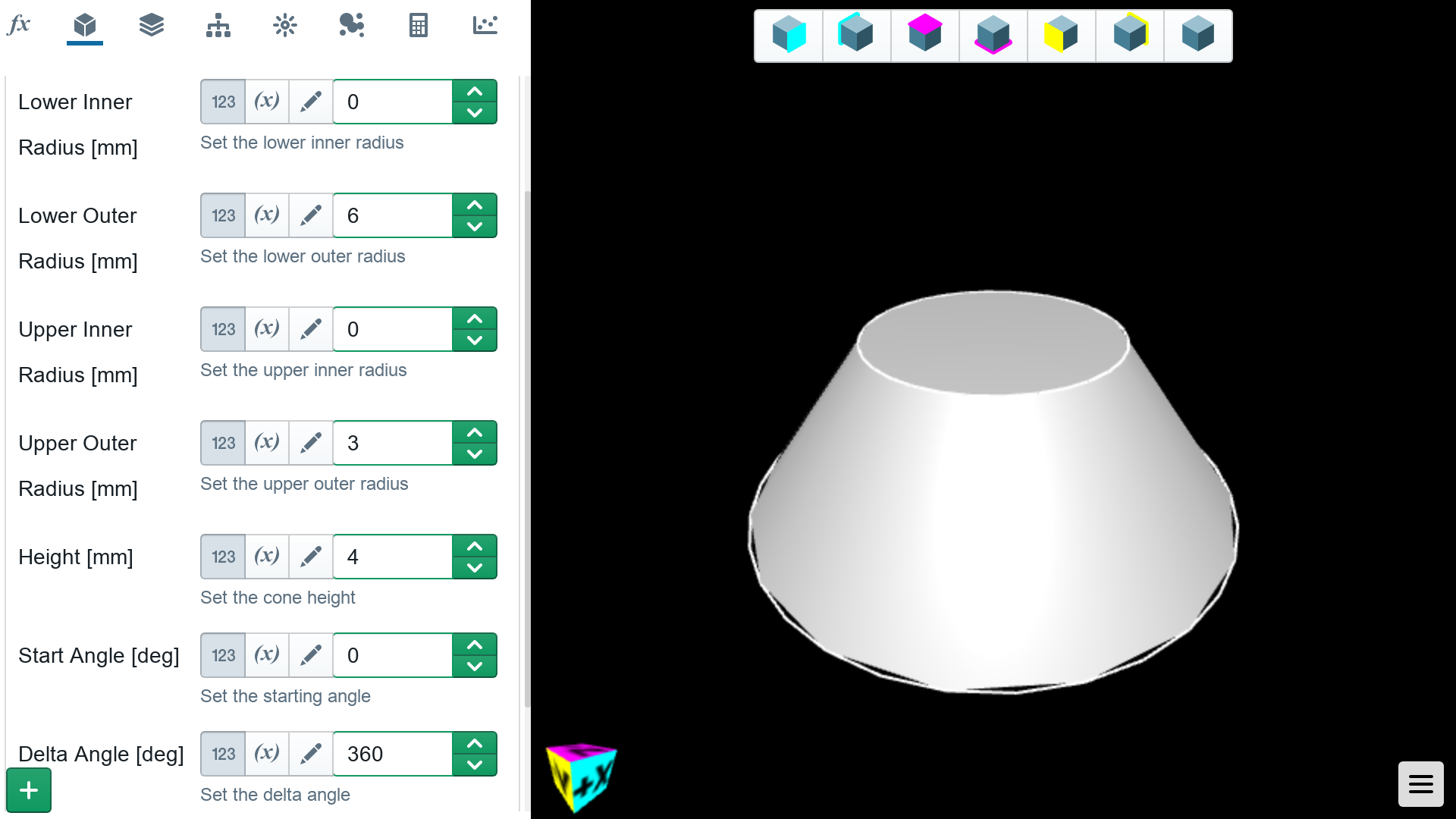Extra parameters allow for hollow cones, and cones partially swept around the vertical axis.

#### Optional Parameters

• `upper outer radius > upper inner radius >= 0`

• `lower outer radius > lower inner radius >= 0`

• `start angle >= 0`

• `2pi >= delta angle > 0`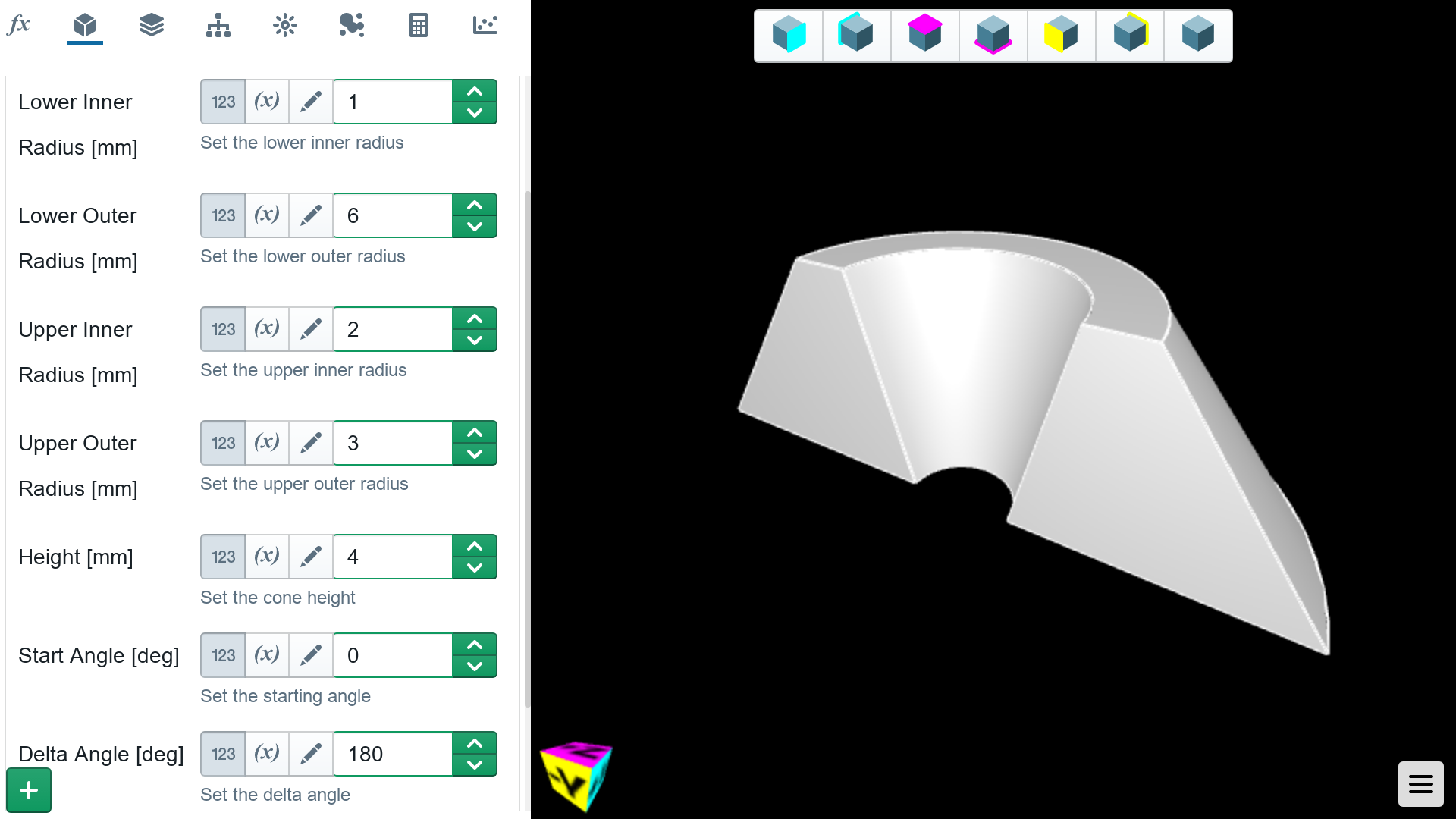## Ellipsoid

An ellipsoid is defined with radii in the three cardinal directions. If all radii are equal, the ellipsoid will be equivalent to a sphere.

#### Parameters

• `x radius > 0`
• `y radius > 0`
• `z radius > 0`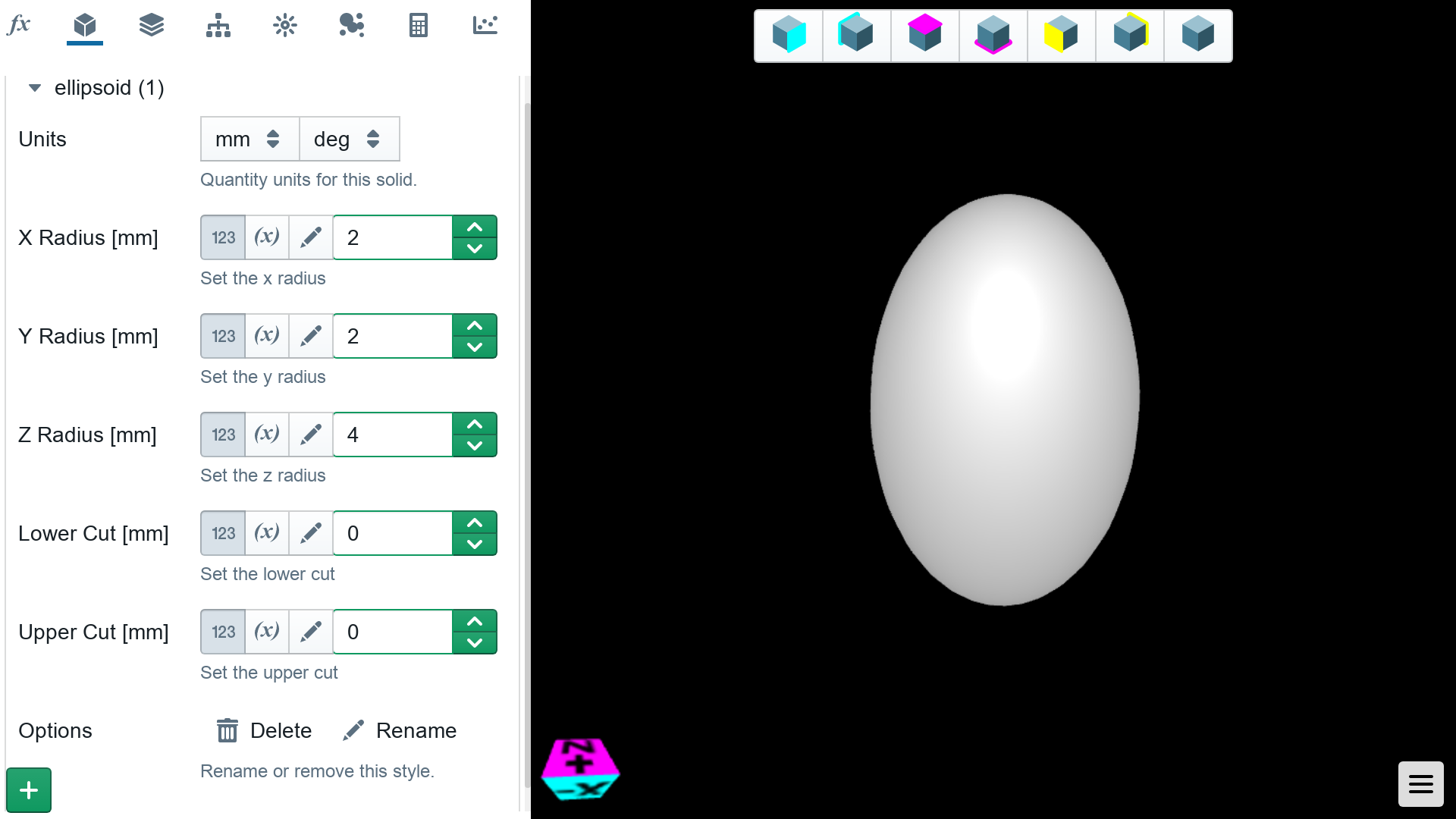Optional parameters allow for cutting the top and bottom off of the ellipsoid. The cuts are measured for the top and bottom of the ellipsoid respectively.

#### Optional Parameters

• `lower cut >= 0` - `upper cut >= 0`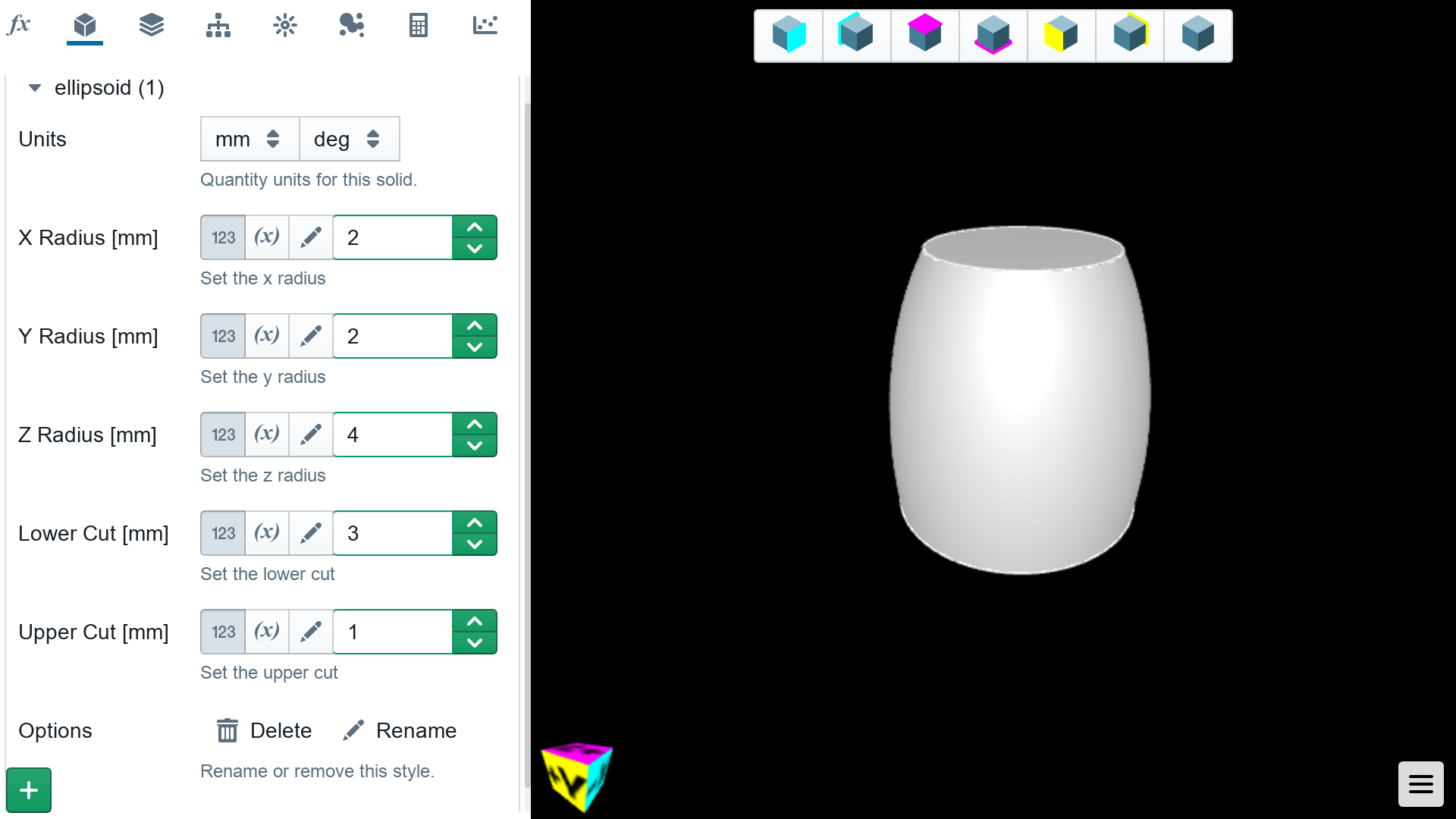## Sphere

The basic sphere is desibred with a single outer radius. A hollow sphere can be created by setting an inner radius.

#### Parameters

• `inner radius >= 0`

• `outer radius > inner radius`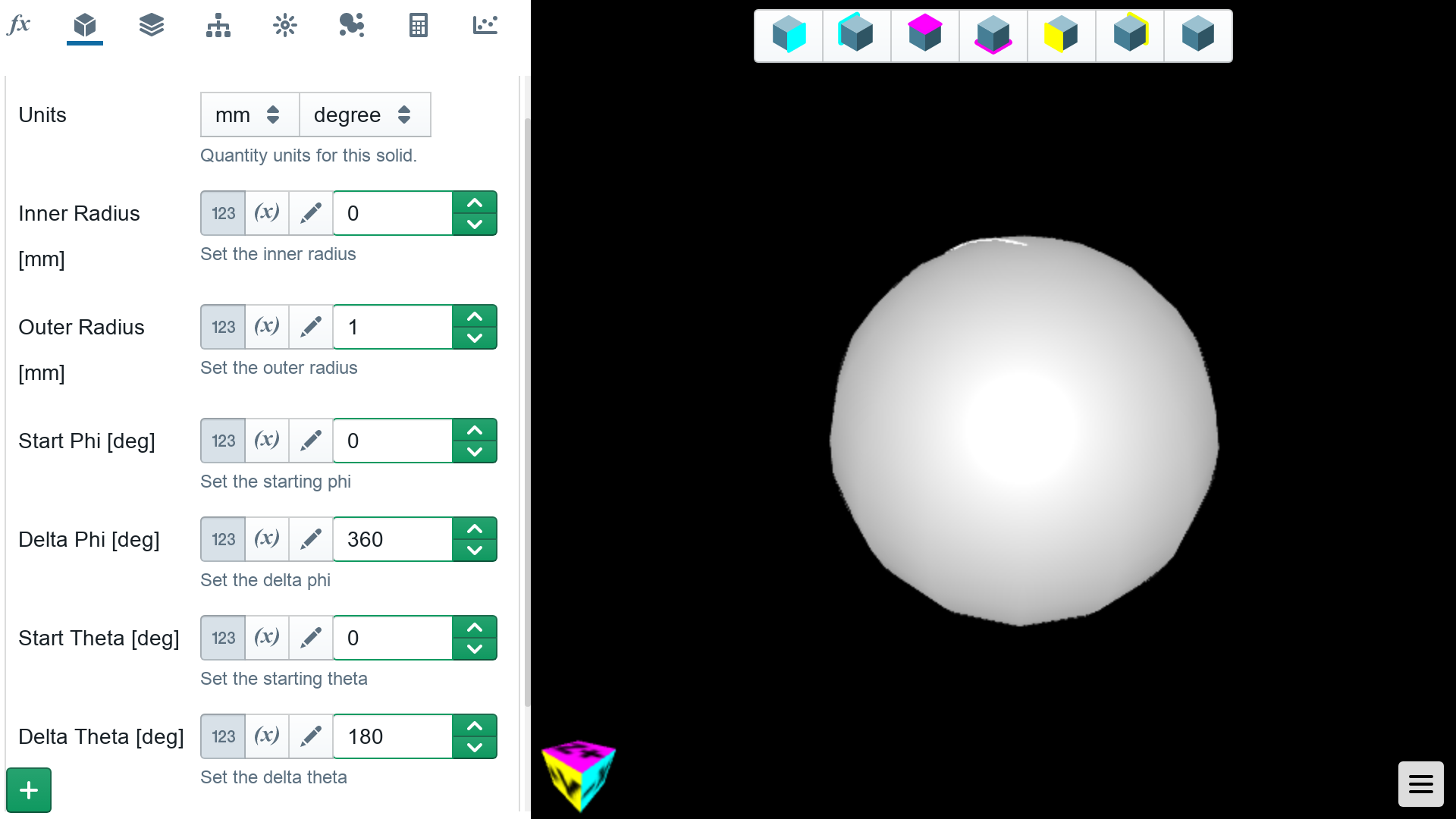The sphere can also be swept in the phi and theta directions allowing for hollow partial spheres.

#### Optional Parameters

• `start phi > 0`

• `2pi >= delta phi > 0`

• `start theta > 0`

• `pi >= delta theta > 0`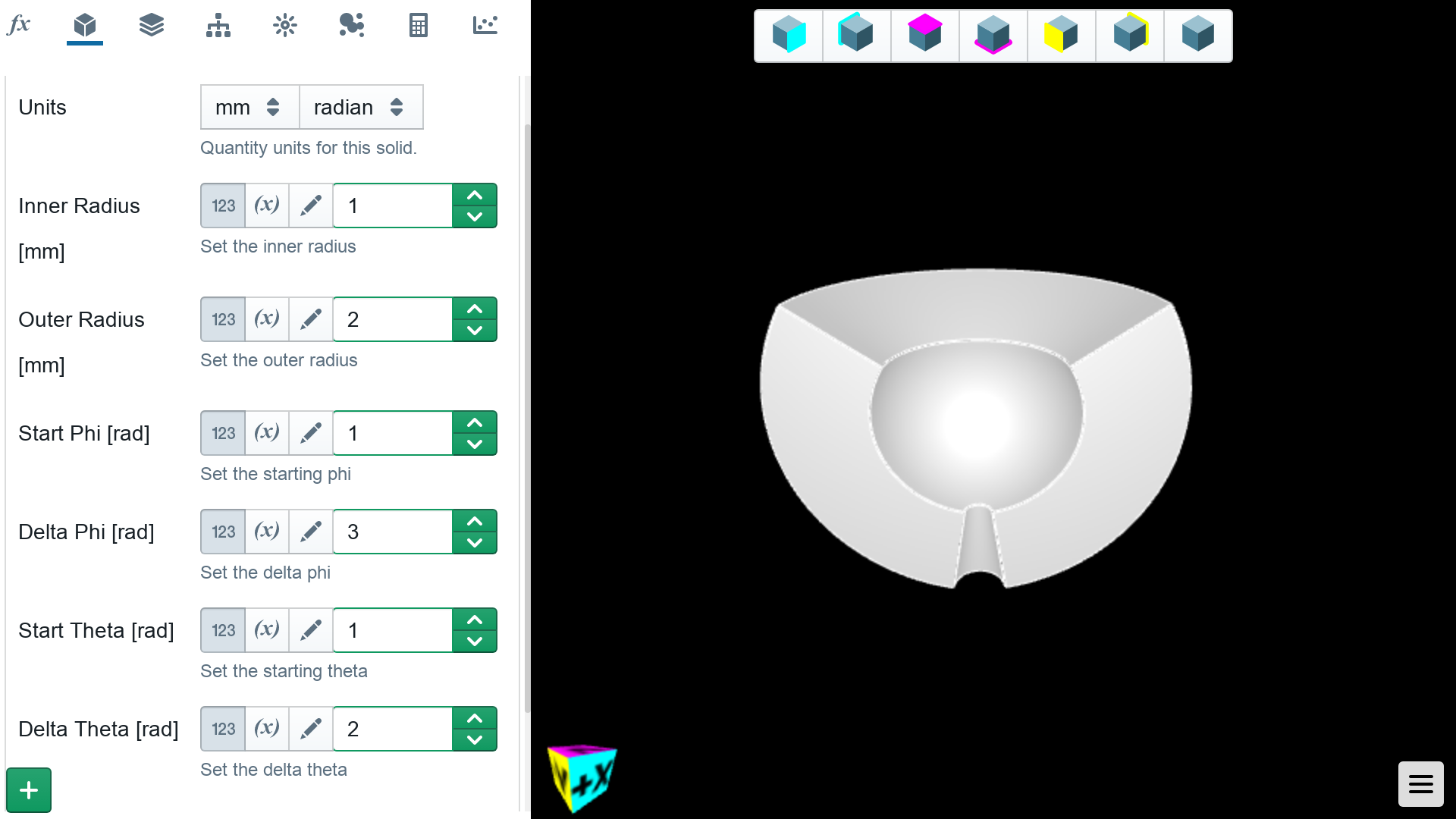## Torus

A torus is a tube with an inner and outer radius, swept around a sweep radius. The torus can be swept around a full or particle circle by setting the start and delta angles.

#### Parameters

• `sweep radius > 0`
• `inner radius >= 0`
• `outer radius > inner radius`
• `start angle >= 0`
• `2pi >= delta angle > 0`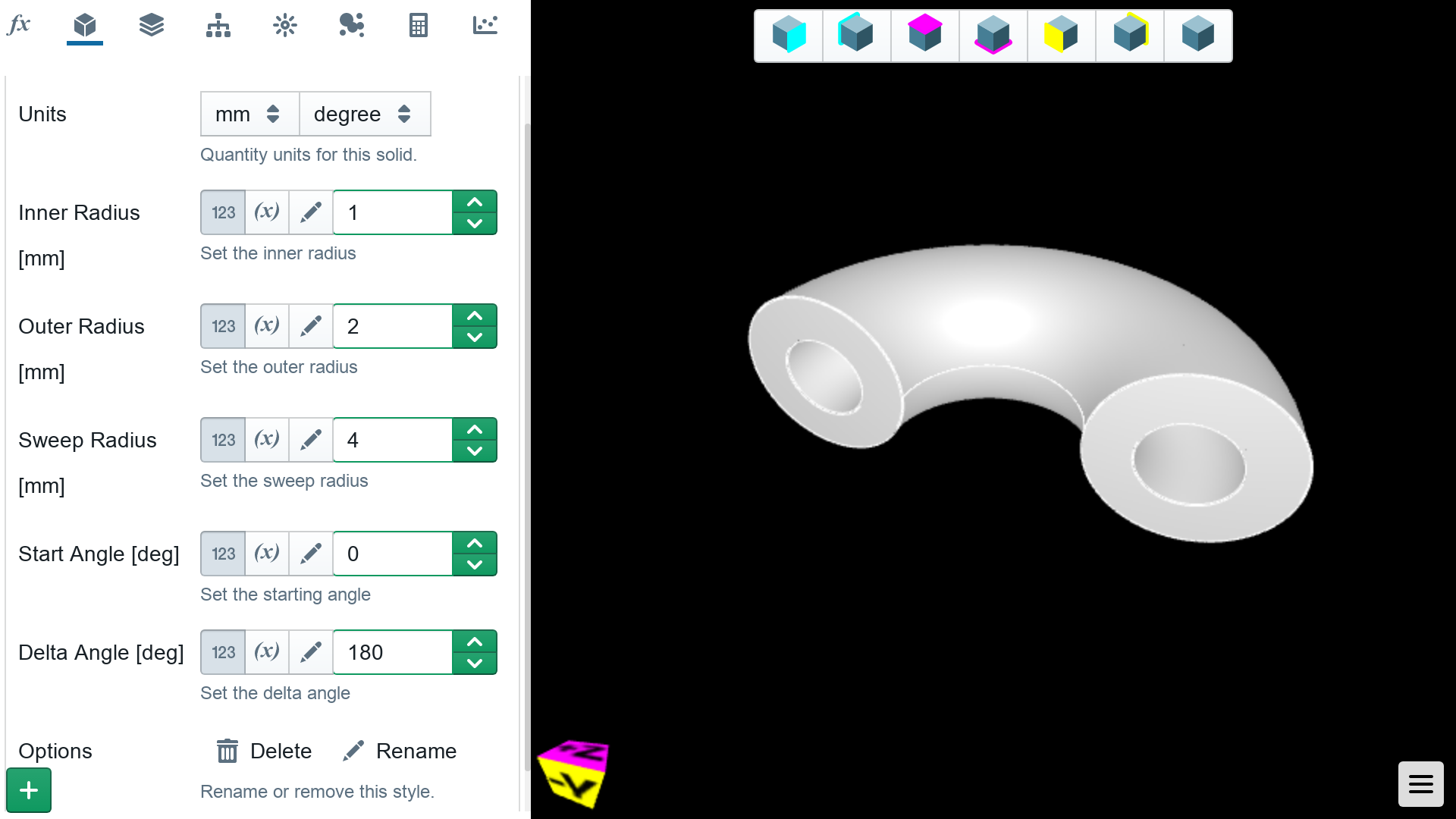## Tube

A cylinder can be defined simply with a height and outer radius, with an inner radius of zero. Tubes can be formed by setting an inner radius. Partial tubes can be swept around the tube axis.

#### Parameters

• `height > 0`
• `inner radius >= 0`
• `start angle >= 0`
• `2pi >= delta angle > 0`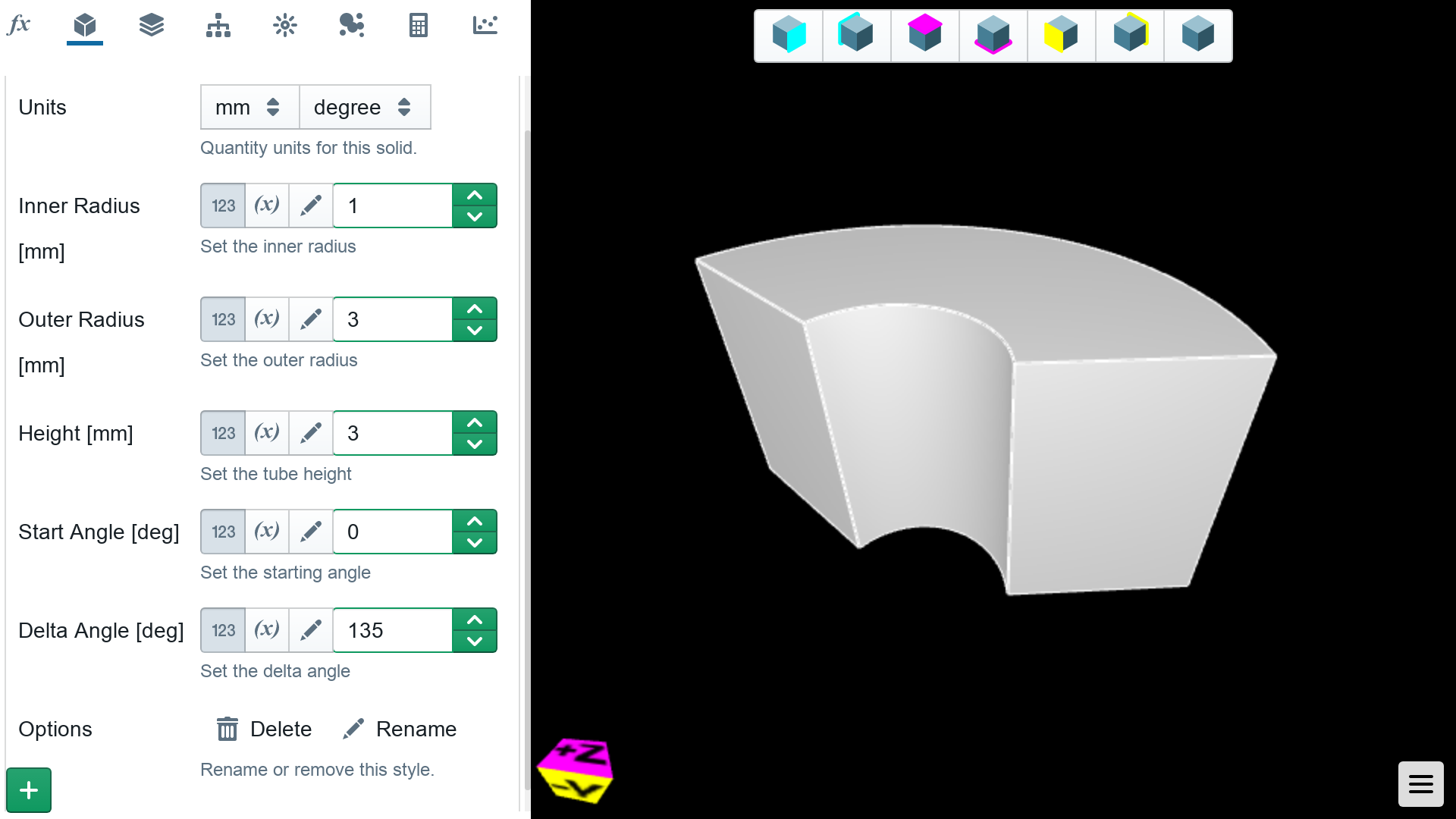CAD files can be loaded into Shielding Studio as triangular meshes. Most popular CAD packages are able to export geometry in text based triangle file formats such as STL and PLY. This are the types of files that can be imported by Shielding Studio.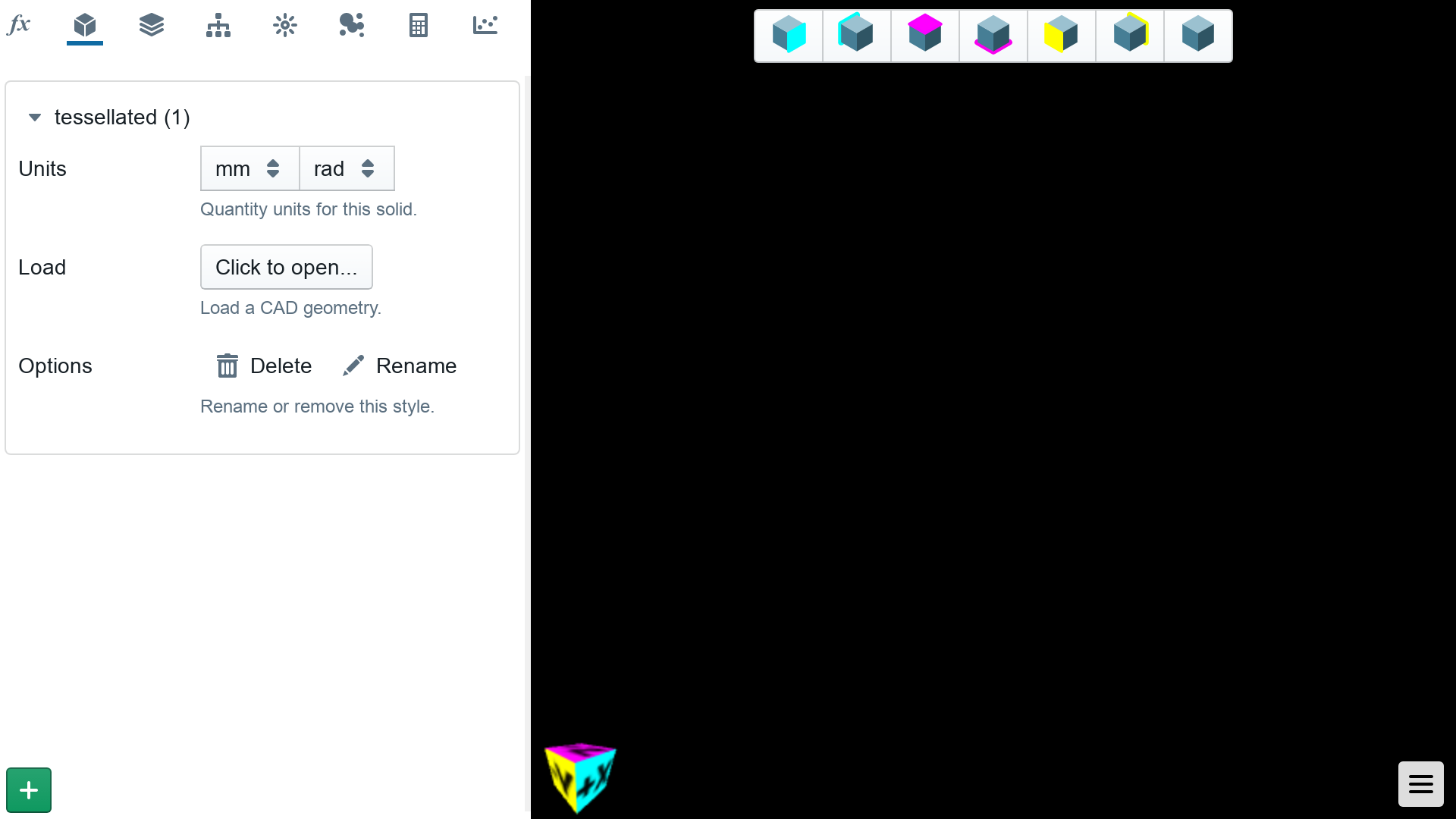When the CAD geometry is first loaded into Shielding Studio, it is positioned in absolute coordinates around the origin defined in the file. This may or may not put the geoemtry around the origin, or in the correct orientation.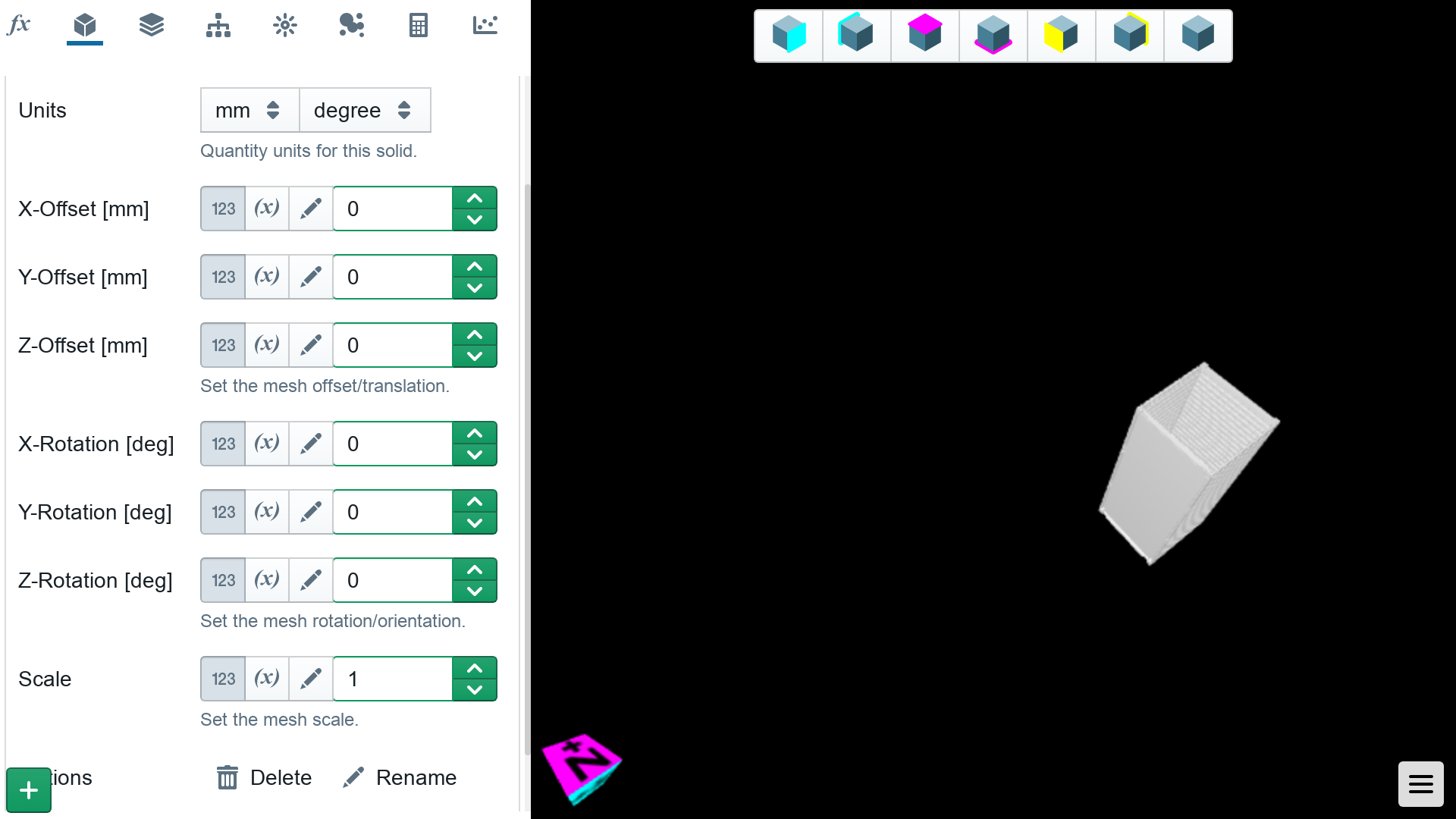The position, orientation, and scale (for setting unit conversions for example) can be set using the parameter controls for the geometry once it has been loaded.